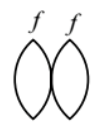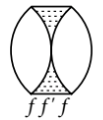Ray optics
Question

# Two similar thin equi-convex lenses, of focal length f each, are kept coaxially in contact with each other such that the focal length of the combination is ${\mathrm{F}}_{1}$. When the space between the two lenses is filled with glycerin (which has the same refractive index $\left(\mathrm{\mu }=1.5\right)$ as that of glass) then the equivalent focal length is ${\mathrm{F}}_{2}$. The ratio ${\mathrm{F}}_{1}$:${\mathrm{F}}_{2}$ will be

Difficult
Solution

## Two similar equi-convex lenses of focal length f each are held in contact with each other.The focal length ${\mathrm{F}}_{1}$ of the combination is given byFor glycerin in between lenses, there are three lenses, one concave and two convexFocal length of the curve lens is given$\frac{1}{{\mathrm{f}}^{\text{'}}}=\left(1.5-1\right)\left(\frac{-2}{\mathrm{R}}\right)=-\frac{1}{\mathrm{R}}$Now, equivalent focal length of the combination is given by

Get Instant Solutions